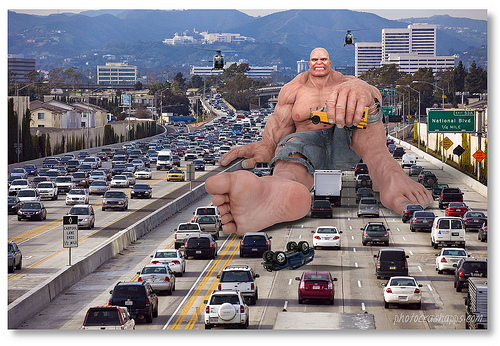# The escape from L.A.

Probability Level 5You're driving from Caltech, in Pasadena, to San Diego after a long day in the lab. You're cruising along and everything is going great until you hit Rosecrans Avenue, a horrible street in horrible Los Angeles. Here, everything grinds to a halt and you sit in mind numbing gridlock for a long time. Bored to tears, you start thinking about your situation and come up with the following simple model.

Let's approximate Rosecrans Ave as a 1d lattice with $n$ locations for cars $x_1,x_2,\ldots,x_n$ each of which can hold only one car. At each moment in time, traffic flow on Rosecrans works as follows:

• If there is no car on the first position on Rosecrans ($x_0$), a new car will enter it at the rate $p_\text{injection}$.
• If there is a car at last position on the avenue ($x_n$), the car will leave Rosecrans (and move on to the highway) at the rate $p_\text{removal}$.
• If there is a car at $x_i$ and no car at $x_{i+1}$, the car on $x_i$ will move to $x_{i+1}$ at the rate $v_\text{car}$.
• If there is a car at $x_i$ and a car at $x_{i+1}$, the car at $x_i$ will stay put.What is the flow rate (on average) of cars leaving Rosecrans?

Assumptions and details

• $p_\text{injection} = 0.6$
• $p_\text{removal} = 0.3$
• $v_\text{car} = 1$
• Assume the street is very long ($n\rightarrow\infty$)
• Give your answer in $\text{cars}/\text{unit time}$
×

Problem Loading...

Note Loading...

Set Loading...